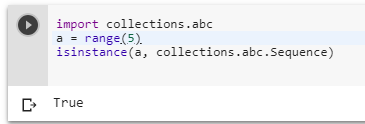3 views
in Python
edited

What makes using range() to make the process of generating a quadrillion values in a instant of time, I am amazed by this can anyone answer this question?

I also tried to run my own function but it didn't helped me:

def my_crappy_range(N):
o = 0
while o< N:
yield o
o += 1
return

by (2k points)
edited by

The reason behind the speed is that in Python 3+ we're using mathematical reasoning about the bounds instead of a direct iteration. It just check all the objects between start and stop and stride value doesn't step over the numbers.

For Ref. you can check the following code:

>>> y, x = 10000000000000, range(10000000000001)
>>> class MyInt(int):
...     pass
...
>>> y_ = MyInt(y)
>>> y in x  # calculates immediately :)
True
>>> y_ in x  # iterates for ages.. :(
^\Quit (core dumped)

Happy Learning.

by (106k points)
edited by
• Due to optimization, it is very easy to compare given integers just with min and max range.
• The reason that range() function is so fast in Python3 is that here we use mathematical reasoning for the bounds, rather than a direct iteration of the range object.
• So for explaining the logic here:-
• Check whether the number is between the start and stop, and
• Check whether the step precision value doesn't go over our number.
• Take an example, 997 is in range(4, 1000, 3) because:

4 <= 997 < 1000, and

(997 - 4) % 3 == 0.

• range()  is a sequence, just like a list,and sequences are very fast to work with, It can be tested by below that range() is a sequence given example:-

import collections.abc

a = range(5)

isinstance(a, collections.abc.Sequence)You can use the following video tutorials to clear all your doubts:-